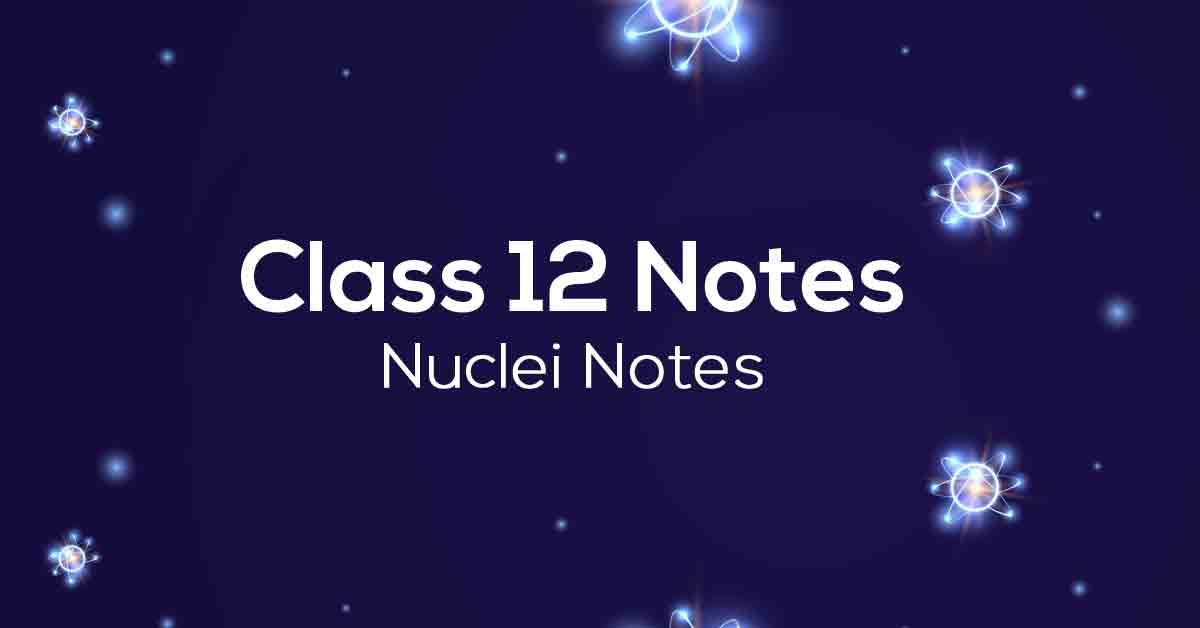Class 12th Physics Nuclei NCERT Notes CBSE 2023

# Nuclei Class 12 Notes# Chapter 13 Nuclei

NCERT Grade 12 Physics Chapter 13 is Nucleus. In this chapter, students will learn about atomic mass and nuclear composition, nuclear size, mass and binding energy, nuclear force, radioactivity, and nuclear energy. It contains the answers to all the questions in the text.

NCERT notes are a great way to prepare for the exam. It will also help students understand the types of questions in competitive exams. Scroll down to read more about Chapter 13 of CBSE Grade 12 Physics.

### Points to Remember

Below we have provided some of the important points to remember for NCERT Nuclei notes for Class 12 Physics to ace your exam:

• An atom has a nucleus. The nucleus is positively charged.

• The nucleus’ radius is smaller than an atom’s radius by a factor of 10 to the power 4. More than 99.9% mass of the atom is concentrated in the nucleus.

• Nuclides with the same atomic number but different neutron numbers are called isotopes.

• Nuclides with the same mass number are isobars.

• Those with the same neutron number are isotones.

• The Q-value of a nuclear process is the amount of energy released or absorbed during the nuclear process.

• In fusion, lighter nuclei combine to form a larger nucleus.

Check out all the important points for CBSE Class 12 Physics Chapter 13 at Vidyakul.

### Topics and Sub- topics

Students can access the Vidyakul learning platform to begin preparing for Year 12 Physics. We provide all content for free.Students can find the list of topics in the NCERT notes for Grade 12 Physics Chapter 13 with exercises in the table below.

 Topic Name Topic Name Atomic Masses And Composition Of Nucleus Beta-decay Size Of The Nucleus Gamma decay Mass-energy And Nuclear Binding Energy Nuclear Energy Mass – Energy Fission Nuclear binding energy Nuclear reactor Nuclear Force Nuclear fusion – energy generation in stars Radioactivity Controlled thermonuclear fusion Alpha decay Law of radioactive decay

### Few Important Questions

• What is an ‘Isotope’?

Isotopes are members of a family of an element that all have the same number of protons but different numbers of neutrons.

• What is ‘Binding energy’?

The energy that holds a nucleus together is called the ‘Binding energy’. This is equal to the mass defect of the nucleus.

### Practice Questions

1. If India had a target of producing by 2021 AD, 500,000 MW of electric power, 10% of which was to be obtained from nuclear power plants. Suppose, the efficiency of utilization (i.e. conversion to electric energy) of thermal energy produced in a reactor was 50%. How much amount of fissionable uranium would our country need per year by 2021? Take the heat energy per fission of U 235 to be about 300 MeV.

1. If Kinetic energy required for one fusion event =average thermal kinetic energy available with the interacting particles = 2(3kT/2); k = Boltzmann’s constant, T = absolute temperature. That is the kinetic energy needed to overcome the Coulomb repulsion between the two nuclei? Mention the temperature up to which the gas be heated to initiate the reaction? Consider the radius of both deuterium and tritium to be approximately 3.0 fm.